Next: Multi-Dimensional Search Up: Minimization in One Previous: Minimization in One

### Golden Section Search

An elegant and robust method of locating a minimum in such a bracket is the Golden Section Search. This involves evaluating the function at some point x in the larger of the two intervals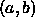or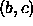. If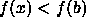then x replaces the midpoint b, and b becomes an end point. If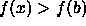then b remains the midpoint with x replacing one of the end points. Either way the width of the bracketing interval will reduce and the position of the minima will be better defined (Figure 2). The procedure is then repeated until the width achieves a desired tolerance. It can be shown that if the new test point, x, is chosen to be a proportion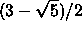(hence Golden Section) along the larger sub-interval, measured from the mid-point b, then the width of the full interval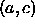will reduce at an optimal rate .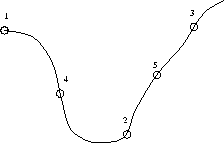Figure 2: Golden Section Search. Initial bracket (1,2,3) becomes (4,2,3), (4,2,5)...

The Golden Section Search requires no information about the derivative of the function. If such information is available it can be used to predict where best to choose the new point x in the above algorithm, leading to faster convergence .

Bob Fisher
Fri Mar 28 14:12:50 GMT 1997